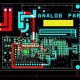# How to Disturb Mutual Inductance in PCB?

In this tutorial we are going to learn about How to Disturb Mutual Inductance in PCB?

If there is two loop of current, there is mutual inductance .The current in one loop creates a magnetic field, and that magnetic field affects the second loop. Generally two lops interact, with the coefficient of interaction decaying rapidly with increasing distance The coefficient of interaction between two loops is called their mutual  inductance , units of which are  volt second/amp a mutual inductive coupling between two circuits acts the same as a tiny transformer connected between circuit A and circuit B as shown in figure 1.18 anywhere we see two nearby loops of current the two currents interact like the primary and secondary of a transformer , and we get mutual inductance

When mutual inductance Lm injects a noise voltage Y into circuit B proportional to the rate of change of current in circuit A according to this rule:

Y= LM DIA/DT

Suddenly changes in current in loop a induce large voltage in loop B, hence the importance of mutual inductive coupling to high-speed design.  It is to the actual coupled noise voltage a complete formula would use the difference in currents between primary and secondary and the loading effect of the primary and secondary  windings on both circuit the imagination  surrounding the use f equation 1.32 are similar to those for equation 1.25 namely :

• The induced voltage across LM is much smaller than the primary signal voltage attaching LM  therefore does not load circuit A .noise voltage coupled by mutual  inductance in digital products are always smaller than the source signal.
• The coupled signal current in circuit B is smaller than the current in A  .We can ignore the small coupled in B and assume the difference between primary and secondary side currents in the coupling transformer just equals IA
• Suppose that the secondary impedance is small compared to the impedance to ground of circuit B just add the coupled noise voltage to the voltage otherwise present in circuit B and due to this procedure ignore interactions between the mutual inductance and the secondary circuit .
• For digital circuits mutual inductance, like mutual capacitance, usually induces unwanted crosstalk between circuits

• When any current in loop A produces a pattern of magnetic field energy. On  stronger currents, more magnetic energy is stored is stored per unit volume in the space surrounding loop A.